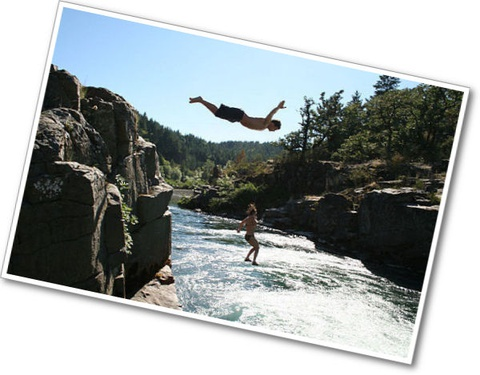# Gallery Problems# Diving

In this diagram, the vertical number line represents distances above and below sea level. Sea level is at 0 meters.

• Chen is standing on top of a cliff that is 20 meters above sea level. Write his position as a positive number on the number line.
• The diver is swimming 10 meters below sea level. Write her position as a negative number on the number line.
• The sea floor is 50 meters below the cliff top. Write this point as a negative number on the number line.
• Suppose the diver swims to a position that is 5 meters above the sea floor. Write her position as a positive or negative number on the number line.1 of 6